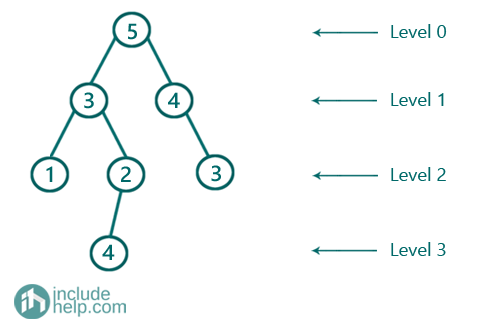# Max Level Sum in Binary Tree

In this article, we are going to see how to find the level having maximum sum using level order traversal?
Submitted by Radib Kar, on September 01, 2020

Here, we are going to find how we can find the level having maximum sum using level order traversal. If you are not familiar with level order traversal then please have a look to the pre-requisite please. To find out the level having maximum sum with need to calculate sum of each level and then need to decide which one has the maximum sum. In the below example,The level sums are:

Level 0-> 5
Level 1-> 7
Level 2-> 6
Level 3-> 4

So the maximum sum is 7 at level 1

The algorithm is:

1. Max isto store the maximum level sum
2. Do a level order traversal & store current sum in max if current sum is greater than max

Below is the detailed implementation:

```#include <bits/stdc++.h>
using namespace std;

// tree node is defined
class TreeNode {
public:
int val;
TreeNode* left;
TreeNode* right;
TreeNode(int data)
{
val = data;
left = NULL;
right = NULL;
}
};

int maxLevelSum(TreeNode* root, int& maxlevel)
{
queue<TreeNode*> q;
//to store sum for a level
int sum = 0;
//max is  to store the maximum level sum
int max = INT_MIN;
int level = 0;
TreeNode* temp;

q.push(root); //first level, root only
q.push(NULL); //end of first level
//while queue is not empty
while (!q.empty()) {
//DeQueu
temp = q.front();
q.pop();
//if end of current level
if (temp == NULL) {
//if queue is not empty place NULL at the end of
//the queue to denote end of upcoming level
if (!q.empty()) {
q.push(NULL);
}
//if current level sum is ? max, update max
if (max < sum) {
max = sum;
maxlevel = level; //stores the level having max sum
}
//since it's end of current level initialize sum as
//0 again to calculate for next level
sum = 0;
level++;
}
else {
sum += temp->val; //find current level sum
//do level order traversal
if (temp->left)
q.push(temp->left);
if (temp->right)
q.push(temp->right);
}
}
return max;
}

int main()
{
//building the tree like the example

TreeNode* root = new TreeNode(5);
root->left = new TreeNode(3);
root->right = new TreeNode(4);
root->left->left = new TreeNode(1);
root->left->right = new TreeNode(2);
root->right->right = new TreeNode(3);
root->left->right->left = new TreeNode(4);
int maxlevel = 0;
cout << "Maximum level sum in the given tree is: " << maxLevelSum(root, maxlevel) << endl;
cout << "And the level having the sum is: " << maxlevel << endl;

return 0;
}
```

Output:

```Maximum level sum in the given tree is: 7
And the level having the sum is: 1
```

Since the above implementation is pretty intuitive, we are not going for any dry run. But if you have any difficulty in understanding, please do comment below and try a dry run from your end.

What's New

Top Interview Coding Problems/Challenges!

Languages: » C » C++ » C++ STL » Java » Data Structure » C#.Net » Android » Kotlin » SQL
Web Technologies: » PHP » Python » JavaScript » CSS » Ajax » Node.js » Web programming/HTML
Solved programs: » C » C++ » DS » Java » C#
Aptitude que. & ans.: » C » C++ » Java » DBMS
Interview que. & ans.: » C » Embedded C » Java » SEO » HR
CS Subjects: » CS Basics » O.S. » Networks » DBMS » Embedded Systems » Cloud Computing
» Machine learning » CS Organizations » Linux » DOS
More: » Articles » Puzzles » News/Updates